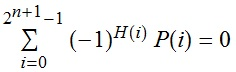# Polynomials and Hamming weights

Let P(x) be a polynomial of degree n. Let H(i) represent the number of `1’s in the binary expansion of the integer i. Although reasonably easy to prove, it may seem surprising that the following identity holds:Does this theorem have a name? I discovered a variation of it years ago when solving a problem given to me by James Aaronson, and applied it again this weekend to reduce an infinite problem to a finite one. One nice corollary of this theorem is that any arithmetic progression of$2^{n+1}$ numbers can be partitioned into two subsets, A and B, such that:

• A and B contain equally many elements;
• The sums of the elements of A and B are equal;
• The sums of squares of elements of A and B are equal;
• (ellipsis)
• The sums of the nth powers of elements of A and B are equal.

By iterative application of this theorem, we can partition an arithmetic progression of$2^{m(n+1)}$ numbers into$2^m$ subsets with this property. This is similar in principle to the idea of a multimagic square, but strictly less impressive.

This entry was posted in Uncategorized. Bookmark the permalink.

### 0 Responses to Polynomials and Hamming weights

1.Maria says:

If it doesn’t have a name, might I suggest ‘Flanelle’s Theorem’? ;P

2.Ross (@rosspresser) says:

I submitted this on math.stackexchange.com . No answer yet, but three upvotes.

http://math.stackexchange.com/questions/467605/does-this-theorem-have-a-name

•Ross (@rosspresser) says:

It now has an answer, but not a name.